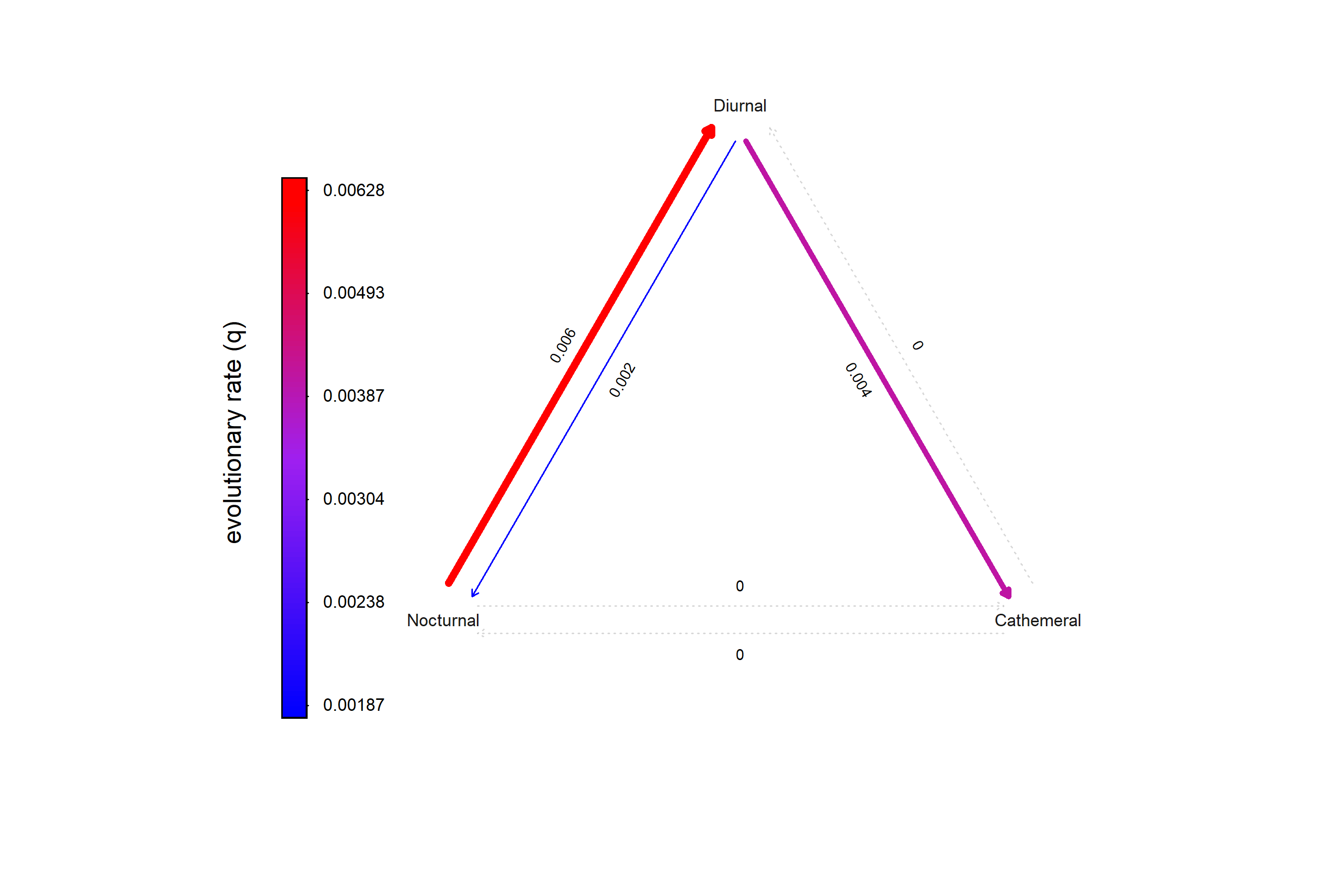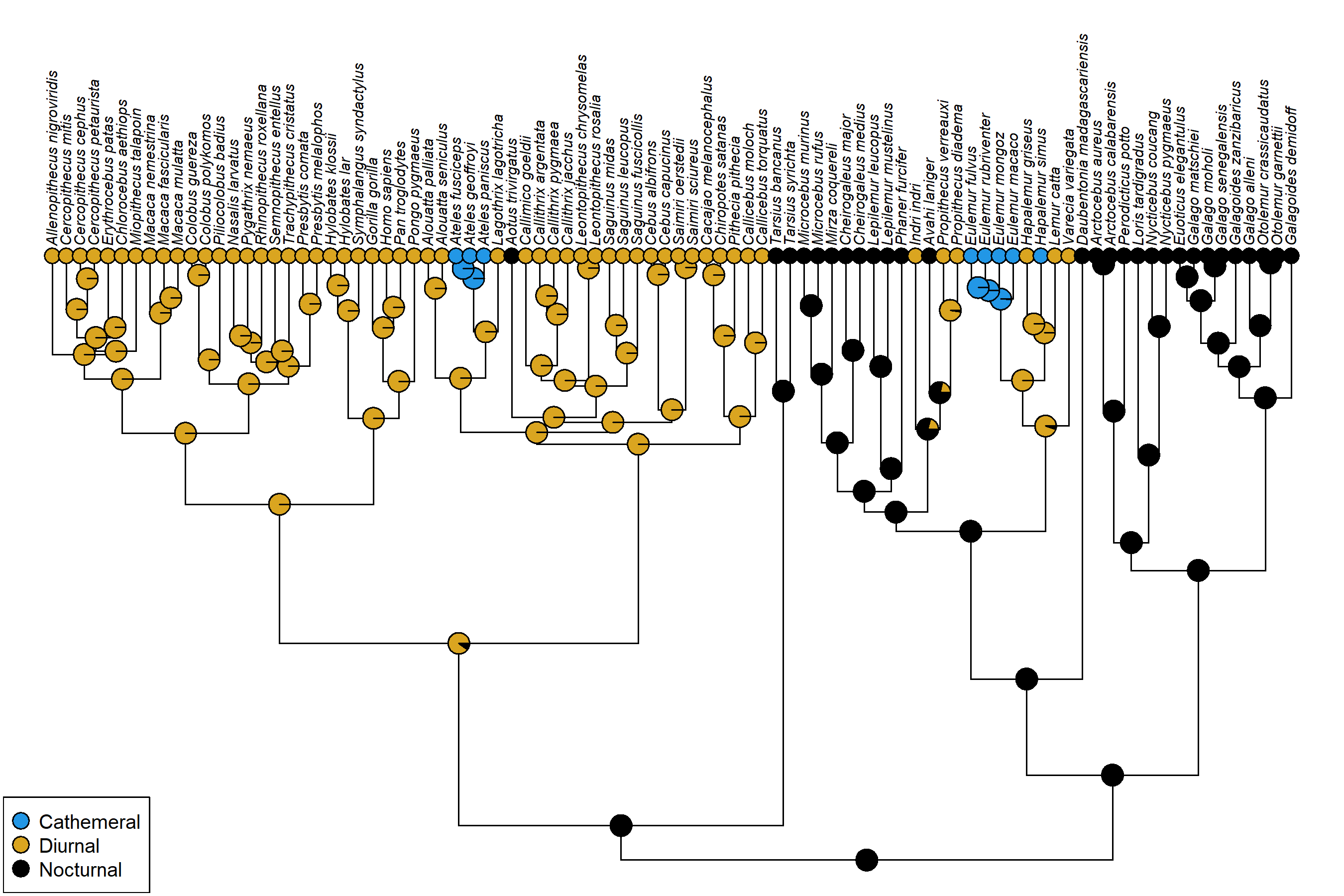## Thursday, March 23, 2023

### Simplified workflow for marginal ancestral state reconstruction using phytools

The following is a very simple workflow for obtaining marginal ancestral states using the new `phytools::ancr` generic method.

To illustrate it, I’ll use diel activity pattern in primates from Kirk & Kay (2004). Readers following along should update phytools from it’s GitHub page.

``````library(phytools)
data(primate.tree)
data(primate.data)
diel_pat<-setNames(as.factor(primate.data\$Activity_pattern),
rownames(primate.data))
``````

Fit chosen discrete character evolution model using `fitMk`.

``````ard_diel<-fitMk(primate.tree,diel_pat,model="ARD",
pi="fitzjohn")
ard_diel
``````
``````## Object of class "fitMk".
##
## Fitted (or set) value of Q:
##            Cathemeral   Diurnal Nocturnal
## Cathemeral    0.00000  0.000000  0.000000
## Diurnal       0.00416 -0.006031  0.001871
## Nocturnal     0.00000  0.006281 -0.006281
##
## Fitted (or set) value of pi:
## Cathemeral    Diurnal  Nocturnal
##   0.000000   0.013462   0.986538
## due to treating the root prior as (a) nuisance.
##
## Log-likelihood: -30.691752
##
## Optimization method used was "nlminb"
``````
``````plot(ard_diel,width=TRUE,color=TRUE,mar=rep(0.1,4),
cex.traits=0.7,offset=0.04)
``````Compute marginal ancestral states for all internal nodes using `ancr`.

``````diel_anc<-ancr(ard_diel)
diel_anc
``````
``````## Marginal ancestral state estimates:
##    Cathemeral  Diurnal Nocturnal
## 91          0 0.000186  0.999814
## 92          0 0.016685  0.983315
## 93          0 0.895729  0.104271
## 94          0 0.996312  0.003688
## 95          0 0.999869  0.000131
## 96          0 0.999985  0.000015
## ...
##
## Log-likelihood = -30.691752
``````

Plot our results!

``````cols<-setNames(c(palette(),"#DAA520","black"),
levels(diel_pat))
plotTree(primate.tree,ftype="i",offset=1,fsize=0.6,
lwd=1,direction="upwards")
nodelabels(pie=diel_anc\$ace,cex=0.4,piecol=cols)
tiplabels(pie=ard_diel\$data[primate.tree\$tip.label,],
cex=0.3,piecol=cols)
legend("bottomleft",names(cols),pch=21,pt.bg=cols,
pt.cex=1.5,cex=0.8)
``````